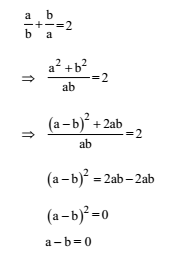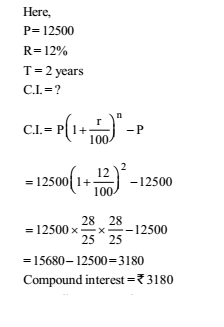# RRB ALP 2018 Practice Test Papers | Arithmetic Questions (Day-37)

Dear Aspirants, Here we have given the Important RRB ALP & Technicians Exam 2018 Practice Test Papers. Candidates those who are preparing for RRB ALP 2018 can practice these Arithmetic Questions to get more confidence to Crack RRB 2018 Examination.

[WpProQuiz 2120]

Click “Start Quiz” to attend these Questions and view Explanation

1. A number x is divisible by 7. When this number is divided by 8, 12 and 16, it leaves a remainder 3 in each case. The least value of x is:

(a) 149

(b) 150

(c) 147

(d) 148

1. A candidate who gets 20% marks in an examination, fails by 30 marks. But if he gets 32% marks, he gets 42 marks more than the minimum pass marks. Find the pass percentage of marks.

(a) 20%

(b) 25%

(c) 12%

(d) 52%

1. P is four times as efficient as Q.P can complete a work in 45 days less than Q. If both of them work together, then in how many days the work will be completed?

(a) 10

(b) 12

(c) 15

(d) 30

1. If a /b+ b /a = 2, then the value of a – b is:

(a) 2

(b) –1

(c) 0

(d) 1

1. The average expenditure of a man for the first five months is Rs. 1200 and for the next seven months is Rs. 1300. If he saves Rs. 2900 in that year, his monthly average income is:

(a) Rs. 1600

(b) Rs. 1700

(c) Rs. 1400

(d) Rs. 1500

1. Of the three numbers, the first is twice the second, and the second is twice the third. The average of the reciprocal of the numbers is 7/12. The numbers are:

(a) 20, 10, 5

(b) 4, 2, 1

(c) 36, 18, 9

(d) 16, 8, 4

1. What is the smallest value that must be added to 91, so that the resultant is a perfect square?

(a) 8

(b) 9

(c) 20

(d) 32

1. If 40% of a number is 290, then what is the number which is 20% more than the initial number?

(a) 870

(b) 725

(c) 825

(d) 680

1. A man bought 15 mangoes for a rupee. How many mangoes were sold for a rupee so that there is a loss of 25%?

(a) 10

(b) 12

(c) 18

(d) 20

1. What is the compound interest (in Rs.) on Rs. 12500 at the rate of 12% per annum compounded yearly for 2 years?

(a) 3000

(b) 2980

(c) 3050

(d) 3180

The least number which leaves a remainder 3 when divided by 8, 12 and 16 is (LCM of 8, 12, 16) k + 3.

LCM of 8, 12, 16 is 48

So least number is 48 k + 3 48 k + 3 is divisible by 7 only when k = 3 So, 48 k + 3 = 48 (3) + 3 = 147

Let total marks on examination = x.

So, minimum passing marks = 20x/100 + 30

20x/100+ 30 = 32 x/100- 42,

After solving, x = 600.

Minimum passing marks =  600*20/100+30 =150

Pass percentage marks 150 /600 x100  = 25%

According to question, If P can complete a work in 1 day,

Q can complete the same work in 4 days.

Hence, if the difference is 3 days, Q can complete the work in 4 days

=> If the difference is 45 days, Q can complete the work in 60 days

Q’s 1 day’s work= 1 /60

P’s 1 day’s work = 4×1/ 60=1/ 15

(P + Q)’s 1 day’s work(1/15+1/60)=1/12

P and Q together can do work in 12 days.

1. Answer: (c)Total expenditure for first 5 months = 5 × 1200 = Rs. 6000

Total expenditure for next 7 months= 7 × 1300 = Rs. 9100

Total saving = Rs. 2900

So, total yearly income = 6000 + 9100 + 2900 = Rs. 18000

Monthly average income = 18000 12 = Rs. 1500

Let third number = x then,

second number = 2x

first number = 4 x

According to question

(1/x+1/2x+1/3x)/3=7//12

x = 9

first number = 4x = 4 × 9 = 36

second number = 2x = 2 × 9 = 18

third number = x = 9

9<√91<10

91+9=100

100  is square of 10.

Let original number = x

According to question, x .40/100=

X= 290 x100/40=725

Required number= 725x 120/100= 870

CP of a mango= 1/ 15

Loss = 25%

SP=1/ 15×75/100=1/20RRB ALP 2018 Practice Test Papers | Arithmetic Questions (Day-1)

RRB ALP 2018 Practice Test Papers | Arithmetic Questions (Day-2)

RRB ALP 2018 Practice Test Papers | Arithmetic Questions (Day-3)

RRB ALP 2018 Practice Test Papers | Arithmetic Questions (Day-4)

RRB ALP 2018 Practice Test Papers | Arithmetic Questions (Day-5)

RRB ALP 2018 Practice Test Papers | Arithmetic Questions (Day-6)

RRB ALP 2018 Practice Test Papers | Arithmetic Questions (Day-7)

RRB ALP 2018 Practice Test Papers | Arithmetic Questions (Day-8)

RRB ALP 2018 Practice Test Papers | Arithmetic Questions (Day-9)

RRB ALP 2018 Practice Test Papers | Arithmetic Questions (Day-10)

RRB ALP 2018 Practice Test Papers | Arithmetic Questions (Day-11)

RRB ALP 2018 Practice Test Papers | Arithmetic Questions (Day-12)

RRB ALP 2018 Practice Test Papers | Arithmetic Questions (Day-13)

RRB ALP 2018 Practice Test Papers | Arithmetic Questions (Day-14)

RRB ALP 2018 Practice Test Papers | Arithmetic Questions (Day-15)

RRB ALP 2018 Practice Test Papers | Arithmetic Questions (Day-16)

RRB ALP 2018 Practice Test Papers | Arithmetic Questions (Day-17)

RRB ALP 2018 Practice Test Papers | Arithmetic Questions (Day-18)

RRB ALP 2018 Practice Test Papers | Arithmetic Questions (Day-19)

RRB ALP 2018 Practice Test Papers | Arithmetic Questions (Day-20)

RRB ALP 2018 Practice Test Papers | Arithmetic Questions (Day-21)

RRB ALP 2018 Practice Test Papers | Arithmetic Questions (Day-22)

RRB ALP 2018 Practice Test Papers | Arithmetic Questions (Day-23)

RRB ALP 2018 Practice Test Papers | Arithmetic Questions (Day-24)

RRB ALP 2018 Practice Test Papers | Arithmetic Questions (Day-25)

RRB ALP 2018 Practice Test Papers | Arithmetic Questions (Day-26)

RRB ALP 2018 Practice Test Papers | Arithmetic Questions (Day-27)

RRB ALP 2018 Practice Test Papers | Arithmetic Questions (Day-28)

RRB ALP 2018 Practice Test Papers | Arithmetic Questions (Day-29)

RRB ALP 2018 Practice Test Papers | Arithmetic Questions (Day-30)

RRB ALP 2018 Practice Test Papers | Arithmetic Questions (Day-31)

RRB ALP 2018 Practice Test Papers | Arithmetic Questions (Day-32)

RRB ALP 2018 Practice Test Papers | Arithmetic Questions (Day-33)

RRB ALP 2018 Practice Test Papers | Arithmetic Questions (Day-34)

RRB ALP 2018 Practice Test Papers | Arithmetic Questions (Day-35)

RRB ALP 2018 Practice Test Papers | Arithmetic Questions (Day-36)